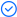WPS Office

Free All-in-One Office Suite with PDF EditorRead, edit, and convert PDFs with the powerful PDF toolkit.Microsoft-like interface, easy to use.

Windows • MacOS • Linux • iOS • Android# How to Use Excel Formula IF Cell Contains Text in Excel

August 1, 2022
21.7K Views

Excel has several formulas that can help you use your data in a convenient way. For example, you can get the output based on whether the cell meets certain specifications. One of Excel's most common tasks is to see if a cell has a value based on a particular condition. This value can be text, date, or any other number.

In this article, we are going to show some Excel formulas to return value in another cell if the cell contains the text.

## Understanding Excel Cells And Text Content

### Cell:

Each worksheet is composed of thousands of rectangles called cells. A cell is made by connecting a row and a column. Columns are identified with letters (A, B, C), and rows with numbers (1, 2, 3).

### Cell content:

Cell content in Excel refers to the information stored within a cell. It can include various types of data, such as text, numbers, dates, formulas, and functions. Cell content can be entered manually or calculated using formulas that reference other cells.

## Excel Formula for Text Content

When working with text content in Excel, you can employ various formulas to achieve specific outcomes. Here are three essential formulas for text content manipulation:

### Using IF Functions in Excel

The IF function is a common Excel function that allows you to perform logical comparisons between a number and what you anticipate.As a result, an IF statement can have two outcomes. If your comparison is True, the first result is True; otherwise, the second result is False.

For example:

=IF(B2=”Yes”,”Done”,”Not yet” ) says IF(B2 = Yes, then return a Done, otherwise return Not yet).

### Using ISNUMBER Function

The ISNUMBER function is helpful when you want to determine if a cell contains a numeric value. This function returns TRUE if the cell contains a number and FALSE if it doesn't. It is particularly useful when you need to differentiate between numeric and non-numeric data in your calculations or logical tests.

For example

Type the formula =ISNUMBER (SEARCH (Yes, B2:B6)) where “YES” is the text to find, B2:B6 is the cell range where you want to find the text.

If you want to add a case-sensitive condition, you must use the FIND function in conjunction with ISNUMBER.

=FIND (find_text, within_text, [start_num])

### Combination of IF-OR/AND-ISNUMBER Function

To create more complex conditions involving text content, you can combine the IF function with the OR and AND functions along with the ISNUMBER function. This allows you to perform multiple logical tests and perform actions based on the outcomes

If you wish to locate “word" or "some" now. You can follow the instructions outlined below.

Enter the formula =IF(OR(ISNUMBER(SEARCH("WINDOWS",A5)), ISNUMBER(SEARCH("DESKTOP,A5)),"AVAILABLE","NOT AVAILABLE"). Where A5 represents the Lookup value

Trustpilot4.8
WPS Office- Free All-in-One Office Suite

• Edit PDF files with the powerful PDF toolkit.

• Microsoft-like interface. Easy to learn. 100% Compatibility.

• Boost your productivity with WPS's abundant free Word, Excel, PPT, and CV templates.

5,820,008 UserAlgirdas Jasaitis

### How does Excel Handle Text Data Differently from Numerical Data?

Excel processes numerical and text data differently. Text data is often regarded as alphanumeric characters and is excluded from mathematical computations. However, numerical data may be employed in mathematical operations such as addition, subtraction, multiplication, and division.

### What are Some Common Mistakes to Avoid When Using the 'IF' Function with Text?

When using the 'IF' function with text, some common mistakes to avoid include

• Not closing the parenthesis at the conclusion of the formula. Each IF function requires a closing parenthesis, and if they are nested, you must close each one. Although Excel will normally show the missing parentheses, it is critical that you double-check your calculation.

• The incorrect comparison operator was used. Use the appropriate operator for your logical_test, such as "=", ">", ">", "", ">=", or "=".

• For text values, do not use double quotes. When utilizing text values in IF formula, remember to wrap them in double quotes.

### Are There Case-sensitive 'IF' Formulas in Excel?

By default, Excel 'IF' formulas are not case-sensitive. This means that when comparing text values, Excel considers uppercase and lowercase letters as the same. However, there are ways to perform case-sensitive comparisons by using additional functions such as 'EXACT' or by employing array formulas.

### Are there any Best Practices to Follow When Using the 'IF' Function with Text Data?

To ensure accuracy and efficiency when using the 'IF' function with text data, it is recommended to:

• Double-check the syntax of your 'IF' formulas, including the correct use of quotation marks and commas.

• Consider case sensitivity and use appropriate functions if needed.

• Use logical operators such as 'AND' or 'OR' to create complex conditions.

• Test your formulas with different scenarios to ensure they produce the desired results.

• Use cell references instead of hard-coded text values to make your formulas more flexible and adaptable.

## Summary

This comprehensive guide explores various Excel formulas for handling text content. It covers the basics of cells and their addresses, and then dives into essential formulas like IF, ISNUMBER, and the combination of IF-OR/AND-ISNUMBER. The guide provides step-by-step instructions and examples to help users understand and apply these formulas effectively.

Whether you're a beginner or an experienced Excel user, mastering these formulas will enhance your ability to manage and manipulate text content in your spreadsheets. With the power of WPS Office, you can leverage these formulas to maximize your productivity and achieve accurate results in your Excel tasks.